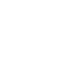# Science Publications: HomeBiological software

Monographs

1. Laser Interaction with Biological Material. Mathematical modeling. Publisher Springer, 2014, 150pp.
2. Mathematical Modeling of Protein Complexes. Springer‐ Nature,2018, Pp.367.
3. Laser Interaction with Heterogeneous Biological Tissue Springer‐Nature,2018, Pp.200.
Publications with peer review process

Koshlan, T.V., Kulikov, K.G. An innovative method for the selection of inhibitors of the viral spike‐glycoprotein of the SARS‐CoV (2021) Journal of Bioinformatics and Computational Biology, 19 (1).

Kulikov, K.G., Koshlan, T.V. Modeling the impact of point mutations on the stability of proteins (2020) Journal of Bioinformatics and Computational Biology, 18 (4).

Koshlan, T.V., Kulikov, K.G. Analysis of Electrostatic Interaction between Dimer Complexes. Part II: Criteria and Conditions on Inhibitors of APP Protein Derivatives(2020) Technical Physics, 65 (8), pp. 1313‐1320.

Koshlan, T.V., Kulikov, K.G. Analysis of Electrostatic Interaction between Dimer Complexes. Part I: A Method for Selecting Inhibitors of APP Protein Derivatives (2020)
Technical Physics, 65 (7), pp. 1167‐1174.

Koshlan, T.V., Kulikov, K.G. Simulation of the Effect of Point Mutations on the Stability of Protein Dimers Using the Bcl‐2 Protein Family as an Example (2020) Technical Physics,
65 (4), pp. 518‐533.

Koshlan, T.V., Kulikov, K.G.Analysis of Electrostatic Interactions of Amino Acid Residues by the Example of Formation of a Nap1–Nap1 Dimer (2020)Technical Physics, 65 (3), pp. 333‐339.

Kulikov, K.G., Koshlan, T.V. Mathematical Modeling of Linear Docking. I. Determination of Regions of Binding of Protein Molecules (2018) Technical Physics, 63 (8), pp. 1101‐1114.

Koshlan, T.V., Kulikov, K.G. Mathematical Modeling of Linear Docking. II. Estimating the Effect of Point Mutations on the Affinity between Protein Molecules (2018)
Technical Physics, 63 (8), pp. 1115‐1124.

Koshlan, T.V., Kulikov, K.G. Mathematical modeling of the temperature effect on the character of linking between monomeric proteins in aqueous solutions (2017)
Technical Physics, 62 (11), pp. 1736‐1743.

Koshlan, T.V., Kulikov, K.G. Mathematical modeling the formation of a histone octamer (2017) Technical Physics, 62 (5), pp. 684‐690.

Koshlan, T.V., Kulikov, K.G. Mathematical simulation of complex formation of protein molecules allowing for their domain structure (2017) Technical Physics, 62 (4), pp. 509‐
516.

Kulikov, K.G., Koshlan, T.V. Mathematical simulation of interactions of protein molecules and prediction of their reactivity(2016) Technical Physics, 61 (10), pp. 1572‐ 1579.

Kulikov, K.G., Koshlan, T.V. Measurement of sizes of colloid particles using dynamic light scattering (2015) Technical Physics,60(12),pp. 1758‐1764.

Kulikov, K.G., Koshlan, T.V. Simulation of the type of coralin alkaloid‐DNA binding (2015) Technical Physics, 60 (5), pp. 639‐644.

Kulikov, K.G. Investigation into the electrophysical characteristics of hemocytes by intracavity laser spectroscopy. II: Numerical simulation (2014) Technical Physics, 59 (5),
pp. 782‐786.

Kulikov, K.G. Study of electrophysical characteristics of blood formed elements using
intracavity laser spectroscopy. I. Simulation of lightscattering by an ensemble of biological cells with complicated structures
(2014) Technical Physics, 59 (4), pp. 576‐587.

Kulikov, K.G. Mathematical simulation of electrophysical characteristics of multiply scattering media with a fibrillar structure. I. Theory and computational model (2013) Technical Physics,58 (11), pp. 1571‐1577.

Kulikov, K.G. Mathematical simulation of electrophysical characteristics of multiply
scattering media with a fibrillar structure. II. Numerical calculations
(2013) Technical
Physics, 58 (11), pp. 1702‐ 1704.

Kulikov, K.G.Light scattering by dielectric bodies of irregular shape in a layered
medium in problems of biomedical optics: I. Theory and computational model
(2012)
Technical Physics, 57 (12), pp. 1623‐1631.

Kulikov, K.G. Light scattering by dielectric bodies of irregular shape in a layered
medium in problems of biomedical optics: II. Numerical analysis
(2012) Technical
Physics, 57 (12), pp. 1632‐1636.

Kulikov, K.G. Simulation of the electrophysical characteristics of a biological tissue
with allowance for large‐scale in homogeneities
(2012) Technical Physics, 57 (7), pp. 907‐ 914.

Kulikov, K.G. Analysis of the effect of small‐scale in homogeneities on the
electrophysical characteristics of a thin layer by intracavity laser spectroscopy
(2011)
Technical Physics, 56 (9), pp. 1302‐1309.

Kulikov, K.G. The modeling of the temperature field formed inside multilayered
biological tissue under laser emission
(2009) Progress in Biomedical Optics and Imaging ‐ Proceedings of SPIE,7373

Kulikov, K.G. Simulation of electrophysical properties of biological tissues by the
intracavity laser spectroscopy method
(2009) Technical Physics, 54 (3), pp. 435‐439.

Kulikov, K.G. Simulation of the thermal processes induced by action of Laser
(2009) Technical Physics, 54 (2), pp. 259‐267.

Kulikov, K.G. The modeling of the temperature field formed inside Multilayered
biological tissue under laser emission
(2009) Optics InfoBase Conference Papers.

Kulikov, K.G., Radin, A.M. An electrodynamics model of the optical Characteristics of
blood and capillary blood flow rate
(2004) Optics and Spectroscopy, 96 (4), pp. 613‐625.

Kulikov, K.G., Radin, A.M. Study of Dispersion and Absorption of an Ensemble of
Spherical Particles Inside an Optical Cavity and New Possibilities of Predicting the Optical
Characteristics of Biological Media by Intracavity Laser Spectroscopy (2002) Optics and Spectroscopy, 92 (2), pp. 199‐206.